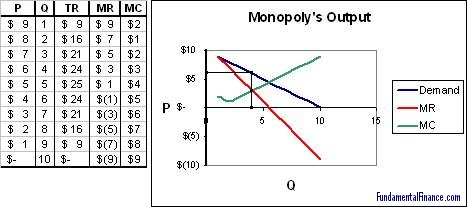## Output

### Output Decision of a Firm

A rational, profit-maximizing firm will choose to produce the quantity where marginal cost is equal to marginal revenue, or where the MC and MR curves intersect. This is true for both competitive firms and monopolies, although the implications are different.

##### Output where: MC = MR

The easiest way (in my opinion) to understand this outcome is by looking at the output levels just before and just after the level where MC = MR. Before this point, marginal revenue is always greater than marginal cost (only in certain exceptions is it not). If a firm is producing at a level where marginal revenue is greater than marginal cost, then by producing one more unit the firm can gain more revenue than it loses in cost and thereby makes a marginal profit. This will be true up to the point that MR = MC. If a firm produces past that point, then marginal revenue is less than marginal cost. This means that the firm is losing profit with each additional unit of output and it should produce less.

To summarize,

• MR > MC: the firm is producing too little and can increase profit by increasing output
• MR < MC: the firm is producing too much and can increase profit by decreasing output

Remember that a competitive firm has a flat MR curve which is equal to the market price. From this we know that a competitive firm will produce at an output where marginal cost equals the market price.

##### A competitive firm produces where: P = MR = MC

A monopoly has a downward sloping MR curve. It will still produce where MR = MC, but at this level price will be higher. This allows the monopoly to make excess profits.In the above table and graph the monopolist would produce at 4 units and charge \$6.

Home
Accounting
Economics
Economics Articles
Economic Data
Economics Glossary
Macroeconomics
Economic Indicators
Fiscal Policy
Microeconomics
The Supply Curve
Price Elasticity
Fixed & Variable Cost
ATC & Marginal Cost
Marginal Revenue
Output Decision
Price Floor
Price Ceiling
Negative Externalities
Positive Externalities
Price Gouging
Sunk Costs
Game Theory
Game Theory Introduction
Nash Equilibrium
Extensive Form
Monopolies
Monopolies
The Sherman Act
The Clayton Act
Papers
Bootstrap Method
The CAPM Model
Euro Creation and Crisis
FX and Inflation in Pakistan
Poverty and Crime
General Finance
Mergers / Acquisitions
Money Market
Options
Real Estate
Retirement
Stocks
Other Personal Finance
Opinions / Essays
Excel